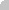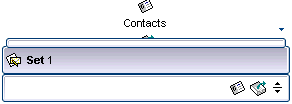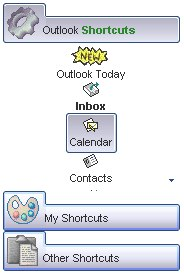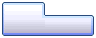method Appearance.Add (ID as Long, Skin as Variant)
Adds or replaces a skin object to the control.Type Description ID as Long A Long expression that indicates the index of the skin being added or replaced. The value must be between 1 and 126, so Appearance collection should holds no more than 126 elements. Skin as Variant A string expression that indicates: an Windows XP Theme part, it should start with "XP:". For instance the "XP:Header 1 2" indicates the part 1 of the Header class in the state 2, in the current Windows XP theme. In this case the format of the Skin parameter should be: "XP: Control/ClassName Part State" where the ClassName defines the window/control class name in the Windows XP Theme, the Part indicates a long expression that defines the part, and the State indicates the state like listed at the end of the document. This option is available only on Windows XP that supports Themes API. a copy of another skin with different coordinates, if it begins with "CP:" . For instance, you may need to display a specified skin on a smaller rectangle. In this case, the string starts with "CP:", and in may looks like "CP:n l t r b", where the n is the identifier being copied, the l, t, r, and b indicates the left, top, right and bottom coordinates being used to adjust the rectangle where the copied skin is displayed. For instance, the "CP:1 4 0 -4 0", indicates that the skin is displayed on a smaller rectangle like follows: Let's say that the control request to paint the {10, 10, 30, 20} area, a rectangle with width of 20 pixels, and height of 10 pixels, the skin will be displayed on the {14,10,26,20} as each coordinates in the "CP" syntax is added to the displayed rectangle. This way you can apply different effects to your objects in your control.the path to the skin file ( *.ebn ). The Exontrol's exButton component installs a skin builder that should be used to create new skins the BASE64 encoded string that holds a skin file ( *.ebn ). Use the Exontrol's exImages tool to build BASE 64 encoded strings on the skin file (*.ebn) you have created. Loading the skin from a file ( eventually uncompressed file ) is always faster then loading from a BASE64 encoded string  A byte[] or safe arrays of VT_I1 or VT_UI1 expression that indicates the content of the EBN file. You can use this option when using the EBN file directly in the resources of the project. For instance, the VB6 provides the LoadResData to get the safe array o bytes for specified resource, while in VB/NET or C# the internal class Resources provides definitions for all files being inserted. ( ResourceManager.GetObject("ebn", resourceCulture) ).Return Description Boolean A Boolean expression that indicates whether the new skin was added or replaced.
Use the Add method to add or replace skins to the control. The skin method, in it's simplest form, uses a single graphic file (*.ebn) assigned to a part of the control. By using a collection of objects laid over the graphic, it is possible to define which sections of the graphic will be used as borders, corners and other possible elements, fixing them to their proper position regardless of the size of the part. Use the Remove method to remove a specific skin from the control. Use the Clear method to remove all skins in the control. Use the BeginUpdate and EndUpdate methods to maintain performance while init the control. Use the Background property to access parts of the control like scroll bar and so on.The identifier you choose for the skin is very important to be used in the background properties like explained bellow. Shortly, the color properties uses 4 bytes ( DWORD, double WORD, and so on ) to hold a RGB value. More than that, the first byte ( most significant byte in the color ) is used only to specify system color. if the first bit in the byte is 1, the rest of bits indicates the index of the system color being used. So, we use the last 7 bits in the high significant byte of the color to indicates the identifier of the skin being used. So, since the 7 bits can cover 127 values, excluding 0, we have 126 possibilities to store an identifier in that byte. This way, a DWORD expression indicates the background color stored in RRGGBB format and the index of the skin ( ID parameter ) in the last 7 bits in the high significant byte of the color. For instance, the BackColor = BackColor Or &H2000000 indicates that we apply the skin with the index 2 using the old color, to the object that BackColor is applied.

The skin method may change the visual appearance for the following parts in the control:

For instance, the following VB sample changes the visual appearance for group headers. The BackColorGroup property indicates the indicates the default group's background color. Shortly, we need to add a skin to the Appearance object using the Add method, and we need to set the last 7 bits in the BackColorGroup property to indicates the index of the skin that we want to use. The sample applies the skin "".

```With ListBar1
.BackColorGroup = &H1000000
End With```

The following C++ sample changes the visual appearance for group headers:

```#include "Appearance.h"
m_listbar.GetVisualAppearance().Add( 1, COleVariant( "D:\\Temp\\ExListBar.Help\\tabup1.ebn" ) );
m_listbar.SetBackColorGroup( 0x1000000 );```

The following VB.NET sample changes the visual appearance for group headers:

```With AxListBar1
.Template = "BackColorGroup = 16777216"
End With```

The following C# sample changes the visual appearance for group headers:

```axListBar1.VisualAppearance.Add(1, "D:\\Temp\\ExListBar.Help\\tabup1.ebn");
axListBar1.Template = "BackColorGroup = 16777216";```

The following VFP sample changes the visual appearance for group headers:

```With thisform.ListBar1
.BackColorGroup = 16777216
EndWith```

where the 16777216 value represents 0x1000000 in hexadecimal.

The screen show was generated using the following template:

On Windows XP, the following table shows how the common controls are broken into parts and states:

Control/ClassNamePartStates
BUTTONBP_CHECKBOX = 3CBS_UNCHECKEDNORMAL = 1 CBS_UNCHECKEDHOT = 2 CBS_UNCHECKEDPRESSED = 3 CBS_UNCHECKEDDISABLED = 4 CBS_CHECKEDNORMAL = 5 CBS_CHECKEDHOT = 6 CBS_CHECKEDPRESSED = 7 CBS_CHECKEDDISABLED = 8 CBS_MIXEDNORMAL = 9 CBS_MIXEDHOT = 10 CBS_MIXEDPRESSED = 11 CBS_MIXEDDISABLED = 12
BP_GROUPBOX = 4GBS_NORMAL = 1 GBS_DISABLED = 2
BP_PUSHBUTTON = 1PBS_NORMAL = 1 PBS_HOT = 2 PBS_PRESSED = 3 PBS_DISABLED = 4 PBS_DEFAULTED = 5
BP_RADIOBUTTON = 2RBS_UNCHECKEDNORMAL = 1 RBS_UNCHECKEDHOT = 2 RBS_UNCHECKEDPRESSED = 3 RBS_UNCHECKEDDISABLED = 4 RBS_CHECKEDNORMAL = 5 RBS_CHECKEDHOT = 6 RBS_CHECKEDPRESSED = 7 RBS_CHECKEDDISABLED = 8
BP_USERBUTTON = 5
CLOCKCLP_TIME = 1CLS_NORMAL = 1
COMBOBOXCP_DROPDOWNBUTTON = 1CBXS_NORMAL = 1 CBXS_HOT = 2 CBXS_PRESSED = 3 CBXS_DISABLED = 4
EDITEP_CARET = 2
EP_EDITTEXT = 1ETS_NORMAL = 1 ETS_HOT = 2 ETS_SELECTED = 3 ETS_DISABLED = 4 ETS_FOCUSED = 5 ETS_READONLY = 6 ETS_ASSIST = 7
EBP_HEADERCLOSE = 2EBHC_NORMAL = 1 EBHC_HOT = 2 EBHC_PRESSED = 3
EBP_HEADERPIN = 3EBHP_NORMAL = 1 EBHP_HOT = 2 EBHP_PRESSED = 3 EBHP_SELECTEDNORMAL = 4 EBHP_SELECTEDHOT = 5 EBHP_SELECTEDPRESSED = 6
EBP_IEBARMENU = 4EBM_NORMAL = 1 EBM_HOT = 2 EBM_PRESSED = 3
EBP_NORMALGROUPBACKGROUND = 5
EBP_NORMALGROUPCOLLAPSE = 6EBNGC_NORMAL = 1 EBNGC_HOT = 2 EBNGC_PRESSED = 3
EBP_NORMALGROUPEXPAND = 7EBNGE_NORMAL = 1 EBNGE_HOT = 2 EBNGE_PRESSED = 3
EBP_SPECIALGROUPBACKGROUND = 9
EBP_SPECIALGROUPCOLLAPSE = 10EBSGC_NORMAL = 1 EBSGC_HOT = 2 EBSGC_PRESSED = 3
EBP_SPECIALGROUPEXPAND = 11EBSGE_NORMAL = 1 EBSGE_HOT = 2 EBSGE_PRESSED = 3
HP_HEADERITEMLEFT = 2HILS_NORMAL = 1 HILS_HOT = 2 HILS_PRESSED = 3
HP_HEADERITEMRIGHT = 3HIRS_NORMAL = 1 HIRS_HOT = 2 HIRS_PRESSED = 3
HP_HEADERSORTARROW = 4HSAS_SORTEDUP = 1 HSAS_SORTEDDOWN = 2
LISTVIEWLVP_EMPTYTEXT = 5
LVP_LISTDETAIL = 3
LVP_LISTGROUP = 2
LVP_LISTITEM = 1LIS_NORMAL = 1 LIS_HOT = 2 LIS_SELECTED = 3 LIS_DISABLED = 4 LIS_SELECTEDNOTFOCUS = 5
LVP_LISTSORTEDDETAIL = 4
MP_MENUBARITEM = 3MS_NORMAL = 1 MS_SELECTED = 2 MS_DEMOTED = 3
MP_CHEVRON = 5MS_NORMAL = 1 MS_SELECTED = 2 MS_DEMOTED = 3
MP_MENUDROPDOWN = 2MS_NORMAL = 1 MS_SELECTED = 2 MS_DEMOTED = 3
MP_MENUITEM = 1MS_NORMAL = 1 MS_SELECTED = 2 MS_DEMOTED = 3
MP_SEPARATOR = 6MS_NORMAL = 1 MS_SELECTED = 2 MS_DEMOTED = 3
MENUBANDMDP_NEWAPPBUTTON = 1MDS_NORMAL = 1 MDS_HOT = 2 MDS_PRESSED = 3 MDS_DISABLED = 4 MDS_CHECKED = 5 MDS_HOTCHECKED = 6
MDP_SEPERATOR = 2
PAGEPGRP_DOWN = 2DNS_NORMAL = 1 DNS_HOT = 2 DNS_PRESSED = 3 DNS_DISABLED = 4
PGRP_DOWNHORZ = 4DNHZS_NORMAL = 1 DNHZS_HOT = 2 DNHZS_PRESSED = 3 DNHZS_DISABLED = 4
PGRP_UP = 1UPS_NORMAL = 1 UPS_HOT = 2 UPS_PRESSED = 3 UPS_DISABLED = 4
PGRP_UPHORZ = 3UPHZS_NORMAL = 1 UPHZS_HOT = 2 UPHZS_PRESSED = 3 UPHZS_DISABLED = 4
PROGRESSPP_BAR = 1
PP_BARVERT = 2
PP_CHUNK = 3
PP_CHUNKVERT = 4
REBARRP_BAND = 3
RP_CHEVRON = 4CHEVS_NORMAL = 1 CHEVS_HOT = 2 CHEVS_PRESSED = 3
RP_CHEVRONVERT = 5
RP_GRIPPER = 1
RP_GRIPPERVERT = 2
SCROLLBARSBP_ARROWBTN = 1ABS_DOWNDISABLED, ABS_DOWNHOT, ABS_DOWNNORMAL, ABS_DOWNPRESSED, ABS_UPDISABLED, ABS_UPHOT, ABS_UPNORMAL, ABS_UPPRESSED, ABS_LEFTDISABLED, ABS_LEFTHOT, ABS_LEFTNORMAL, ABS_LEFTPRESSED, ABS_RIGHTDISABLED, ABS_RIGHTHOT, ABS_RIGHTNORMAL, ABS_RIGHTPRESSED
SBP_GRIPPERHORZ = 8
SBP_GRIPPERVERT = 9
SBP_LOWERTRACKHORZ = 4SCRBS_NORMAL = 1 SCRBS_HOT = 2 SCRBS_PRESSED = 3 SCRBS_DISABLED = 4
SBP_LOWERTRACKVERT = 6SCRBS_NORMAL = 1 SCRBS_HOT = 2 SCRBS_PRESSED = 3 SCRBS_DISABLED = 4
SBP_THUMBBTNHORZ = 2SCRBS_NORMAL = 1 SCRBS_HOT = 2 SCRBS_PRESSED = 3 SCRBS_DISABLED = 4
SBP_THUMBBTNVERT = 3SCRBS_NORMAL = 1 SCRBS_HOT = 2 SCRBS_PRESSED = 3 SCRBS_DISABLED = 4
SBP_UPPERTRACKHORZ = 5SCRBS_NORMAL = 1 SCRBS_HOT = 2 SCRBS_PRESSED = 3 SCRBS_DISABLED = 4
SBP_UPPERTRACKVERT = 7SCRBS_NORMAL = 1 SCRBS_HOT = 2 SCRBS_PRESSED = 3 SCRBS_DISABLED = 4
SBP_SIZEBOX = 10SZB_RIGHTALIGN = 1 SZB_LEFTALIGN = 2
SPINSPNP_DOWN = 2DNS_NORMAL = 1 DNS_HOT = 2 DNS_PRESSED = 3 DNS_DISABLED = 4
SPNP_DOWNHORZ = 4DNHZS_NORMAL = 1 DNHZS_HOT = 2 DNHZS_PRESSED = 3 DNHZS_DISABLED = 4
SPNP_UP = 1UPS_NORMAL = 1 UPS_HOT = 2 UPS_PRESSED = 3 UPS_DISABLED = 4
SPNP_UPHORZ = 3UPHZS_NORMAL = 1 UPHZS_HOT = 2 UPHZS_PRESSED = 3 UPHZS_DISABLED = 4
STARTPANELSPP_LOGOFF = 8
SPP_LOGOFFBUTTONS = 9SPLS_NORMAL = 1 SPLS_HOT = 2 SPLS_PRESSED = 3
SPP_MOREPROGRAMS = 2
SPP_MOREPROGRAMSARROW = 3SPS_NORMAL = 1 SPS_HOT = 2 SPS_PRESSED = 3
SPP_PLACESLIST = 6
SPP_PLACESLISTSEPARATOR = 7
SPP_PREVIEW = 11
SPP_PROGLIST = 4
SPP_PROGLISTSEPARATOR = 5
SPP_USERPANE = 1
SPP_USERPICTURE = 10
STATUSSP_GRIPPER = 3
SP_PANE = 1
SP_GRIPPERPANE = 2
TABTABP_BODY = 10
TABP_PANE = 9
TABP_TABITEM = 1TIS_NORMAL = 1 TIS_HOT = 2 TIS_SELECTED = 3 TIS_DISABLED = 4 TIS_FOCUSED = 5
TABP_TABITEMBOTHEDGE = 4TIBES_NORMAL = 1 TIBES_HOT = 2 TIBES_SELECTED = 3 TIBES_DISABLED = 4 TIBES_FOCUSED = 5
TABP_TABITEMLEFTEDGE = 2TILES_NORMAL = 1 TILES_HOT = 2 TILES_SELECTED = 3 TILES_DISABLED = 4 TILES_FOCUSED = 5
TABP_TABITEMRIGHTEDGE = 3TIRES_NORMAL = 1 TIRES_HOT = 2 TIRES_SELECTED = 3 TIRES_DISABLED = 4 TIRES_FOCUSED = 5
TABP_TOPTABITEM = 5TTIS_NORMAL = 1 TTIS_HOT = 2 TTIS_SELECTED = 3 TTIS_DISABLED = 4 TTIS_FOCUSED = 5
TABP_TOPTABITEMBOTHEDGE = 8TTIBES_NORMAL = 1 TTIBES_HOT = 2 TTIBES_SELECTED = 3 TTIBES_DISABLED = 4 TTIBES_FOCUSED = 5
TABP_TOPTABITEMLEFTEDGE = 6TTILES_NORMAL = 1 TTILES_HOT = 2 TTILES_SELECTED = 3 TTILES_DISABLED = 4 TTILES_FOCUSED = 5
TABP_TOPTABITEMRIGHTEDGE = 7TTIRES_NORMAL = 1 TTIRES_HOT = 2 TTIRES_SELECTED = 3 TTIRES_DISABLED = 4 TTIRES_FOCUSED = 5
TDP_FLASHBUTTON = 2
TBP_BACKGROUNDLEFT = 4
TBP_BACKGROUNDRIGHT = 2
TBP_BACKGROUNDTOP = 3
TBP_SIZINGBARBOTTOM = 5
TBP_SIZINGBARBOTTOMLEFT = 8
TBP_SIZINGBARRIGHT = 6
TBP_SIZINGBARTOP = 7
TOOLBARTP_BUTTON = 1TS_NORMAL = 1 TS_HOT = 2 TS_PRESSED = 3 TS_DISABLED = 4 TS_CHECKED = 5 TS_HOTCHECKED = 6
TP_DROPDOWNBUTTON = 2TS_NORMAL = 1 TS_HOT = 2 TS_PRESSED = 3 TS_DISABLED = 4 TS_CHECKED = 5 TS_HOTCHECKED = 6
TP_SPLITBUTTON = 3TS_NORMAL = 1 TS_HOT = 2 TS_PRESSED = 3 TS_DISABLED = 4 TS_CHECKED = 5 TS_HOTCHECKED = 6
TP_SPLITBUTTONDROPDOWN = 4TS_NORMAL = 1 TS_HOT = 2 TS_PRESSED = 3 TS_DISABLED = 4 TS_CHECKED = 5 TS_HOTCHECKED = 6
TP_SEPARATOR = 5TS_NORMAL = 1 TS_HOT = 2 TS_PRESSED = 3 TS_DISABLED = 4 TS_CHECKED = 5 TS_HOTCHECKED = 6
TP_SEPARATORVERT = 6TS_NORMAL = 1 TS_HOT = 2 TS_PRESSED = 3 TS_DISABLED = 4 TS_CHECKED = 5 TS_HOTCHECKED = 6
TOOLTIPTTP_BALLOON = 3TTBS_NORMAL = 1 TTBS_LINK = 2
TTP_BALLOONTITLE = 4TTBS_NORMAL = 1 TTBS_LINK = 2
TTP_CLOSE = 5TTCS_NORMAL = 1 TTCS_HOT = 2 TTCS_PRESSED = 3
TTP_STANDARD = 1TTSS_NORMAL = 1 TTSS_LINK = 2
TTP_STANDARDTITLE = 2TTSS_NORMAL = 1 TTSS_LINK = 2
TRACKBARTKP_THUMB = 3TUS_NORMAL = 1 TUS_HOT = 2 TUS_PRESSED = 3 TUS_FOCUSED = 4 TUS_DISABLED = 5
TKP_THUMBBOTTOM = 4TUBS_NORMAL = 1 TUBS_HOT = 2 TUBS_PRESSED = 3 TUBS_FOCUSED = 4 TUBS_DISABLED = 5
TKP_THUMBLEFT = 7TUVLS_NORMAL = 1 TUVLS_HOT = 2 TUVLS_PRESSED = 3 TUVLS_FOCUSED = 4 TUVLS_DISABLED = 5
TKP_THUMBRIGHT = 8TUVRS_NORMAL = 1 TUVRS_HOT = 2 TUVRS_PRESSED = 3 TUVRS_FOCUSED = 4 TUVRS_DISABLED = 5
TKP_THUMBTOP = 5TUTS_NORMAL = 1 TUTS_HOT = 2 TUTS_PRESSED = 3 TUTS_FOCUSED = 4 TUTS_DISABLED = 5
TKP_THUMBVERT = 6TUVS_NORMAL = 1 TUVS_HOT = 2 TUVS_PRESSED = 3 TUVS_FOCUSED = 4 TUVS_DISABLED = 5
TKP_TICS = 9TSS_NORMAL = 1
TKP_TICSVERT = 10TSVS_NORMAL = 1
TKP_TRACK = 1TRS_NORMAL = 1
TKP_TRACKVERT = 2TRVS_NORMAL = 1
TRAYNOTIFYTNP_ANIMBACKGROUND = 2
TNP_BACKGROUND = 1
TREEVIEWTVP_BRANCH = 3
TVP_GLYPH = 2GLPS_CLOSED = 1 GLPS_OPENED = 2
TVP_TREEITEM = 1TREIS_NORMAL = 1 TREIS_HOT = 2 TREIS_SELECTED = 3 TREIS_DISABLED = 4 TREIS_SELECTEDNOTFOCUS = 5
WINDOWWP_CAPTION = 1CS_ACTIVE = 1 CS_INACTIVE = 2 CS_DISABLED = 3
WP_CAPTIONSIZINGTEMPLATE = 30
WP_CLOSEBUTTON = 18CBS_NORMAL = 1 CBS_HOT = 2 CBS_PUSHED = 3 CBS_DISABLED = 4
WP_DIALOG = 29
WP_FRAMEBOTTOM = 9FS_ACTIVE = 1 FS_INACTIVE = 2
WP_FRAMEBOTTOMSIZINGTEMPLATE = 36
WP_FRAMELEFT = 7FS_ACTIVE = 1 FS_INACTIVE = 2
WP_FRAMELEFTSIZINGTEMPLATE = 32
WP_FRAMERIGHT = 8FS_ACTIVE = 1 FS_INACTIVE = 2
WP_FRAMERIGHTSIZINGTEMPLATE = 34
WP_HELPBUTTON = 23HBS_NORMAL = 1 HBS_HOT = 2 HBS_PUSHED = 3 HBS_DISABLED = 4
WP_HORZSCROLL = 25HSS_NORMAL = 1 HSS_HOT = 2 HSS_PUSHED = 3 HSS_DISABLED = 4
WP_HORZTHUMB = 26HTS_NORMAL = 1 HTS_HOT = 2 HTS_PUSHED = 3 HTS_DISABLED = 4
WP_MAX_BUTTONMAXBS_NORMAL = 1 MAXBS_HOT = 2 MAXBS_PUSHED = 3 MAXBS_DISABLED = 4
WP_MAXCAPTION = 5MXCS_ACTIVE = 1 MXCS_INACTIVE = 2 MXCS_DISABLED = 3
WP_MDICLOSEBUTTON = 20CBS_NORMAL = 1 CBS_HOT = 2 CBS_PUSHED = 3 CBS_DISABLED = 4
WP_MDIHELPBUTTON = 24HBS_NORMAL = 1 HBS_HOT = 2 HBS_PUSHED = 3 HBS_DISABLED = 4
WP_MDIMINBUTTON = 16MINBS_NORMAL = 1 MINBS_HOT = 2 MINBS_PUSHED = 3 MINBS_DISABLED = 4
WP_MDIRESTOREBUTTON = 22RBS_NORMAL = 1 RBS_HOT = 2 RBS_PUSHED = 3 RBS_DISABLED = 4
WP_MDISYSBUTTON = 14SBS_NORMAL = 1 SBS_HOT = 2 SBS_PUSHED = 3 SBS_DISABLED = 4
WP_MINBUTTON = 15MINBS_NORMAL = 1 MINBS_HOT = 2 MINBS_PUSHED = 3 MINBS_DISABLED = 4
WP_MINCAPTION = 3MNCS_ACTIVE = 1 MNCS_INACTIVE = 2 MNCS_DISABLED = 3
WP_RESTOREBUTTON = 21RBS_NORMAL = 1 RBS_HOT = 2 RBS_PUSHED = 3 RBS_DISABLED = 4
WP_SMALLCAPTION = 2CS_ACTIVE = 1 CS_INACTIVE = 2 CS_DISABLED = 3
WP_SMALLCAPTIONSIZINGTEMPLATE = 31
WP_SMALLCLOSEBUTTON = 19CBS_NORMAL = 1 CBS_HOT = 2 CBS_PUSHED = 3 CBS_DISABLED = 4
WP_SMALLFRAMEBOTTOM = 12FS_ACTIVE = 1 FS_INACTIVE = 2
WP_SMALLFRAMEBOTTOMSIZINGTEMPLATE = 37
WP_SMALLFRAMELEFT = 10FS_ACTIVE = 1 FS_INACTIVE = 2
WP_SMALLFRAMELEFTSIZINGTEMPLATE = 33
WP_SMALLFRAMERIGHT = 11FS_ACTIVE = 1 FS_INACTIVE = 2
WP_SMALLFRAMERIGHTSIZINGTEMPLATE = 35
WP_SMALLHELPBUTTONHBS_NORMAL = 1 HBS_HOT = 2 HBS_PUSHED = 3 HBS_DISABLED = 4
WP_SMALLMAXBUTTONMAXBS_NORMAL = 1 MAXBS_HOT = 2 MAXBS_PUSHED = 3 MAXBS_DISABLED = 4
WP_SMALLMAXCAPTION = 6MXCS_ACTIVE = 1 MXCS_INACTIVE = 2 MXCS_DISABLED = 3
WP_SMALLMINCAPTION = 4MNCS_ACTIVE = 1 MNCS_INACTIVE = 2 MNCS_DISABLED = 3
WP_SMALLRESTOREBUTTONRBS_NORMAL = 1 RBS_HOT = 2 RBS_PUSHED = 3 RBS_DISABLED = 4
WP_SMALLSYSBUTTONSBS_NORMAL = 1 SBS_HOT = 2 SBS_PUSHED = 3 SBS_DISABLED = 4
WP_SYSBUTTON = 13SBS_NORMAL = 1 SBS_HOT = 2 SBS_PUSHED = 3 SBS_DISABLED = 4
WP_VERTSCROLL = 27VSS_NORMAL = 1 VSS_HOT = 2 VSS_PUSHED = 3 VSS_DISABLED = 4
WP_VERTTHUMB = 28VTS_NORMAL = 1 VTS_HOT = 2 VTS_PUSHED = 3 VTS_DISABLED = 4